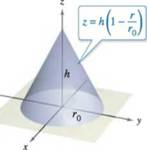Chapter 14.7, Problem 23E

Chapter
Section
Textbook Problem

Using Cylindrical Coordinates In Exercises 23-28, use cylindrical coordinates to find the indicated characteristic of the cone shown in the figure.Find the volume of the cone.

To determine

To calculate: The volume of Cone the equation of the cone is z=h(1rr0)

Explanation

Given:

The provided figure of cone is:

Formula Used:

Volume of cone by triple integration is:

V=dVV=rdzdrdθ

Rectangular conversion equations of cylindrical coordinates:

x=rcosθy=rsinθz=z

Calculation:

Consider the provided figure:

From the cone figure:

Bounds on z are

0zh(1rr0)

Here, h and r0 are constant

Bounds on r are

0rr0

Bounds on θ are

0θ2π

Volume of the cone is

V=dV=02π0r00h(1rr0)rdzdrdθ=02π0r0[rz]0h(1rr0)drdθ=02π0r0rh(1rr0)drdθ

On further integration

<

Still sussing out bartleby?

Check out a sample textbook solution.

See a sample solution

The Solution to Your Study Problems

Bartleby provides explanations to thousands of textbook problems written by our experts, many with advanced degrees!

Get Started

Evaluate limx1x31x1

Calculus: Early Transcendentals

In Exercises 516, evaluate the given quantity. log218

Finite Mathematics and Applied Calculus (MindTap Course List)

Domain Find the domain of the expression. 11. x+3

Precalculus: Mathematics for Calculus (Standalone Book)

Given that the point P(3, 5) lies on the line kx + 3y + 9 = 0, find k.

Applied Calculus for the Managerial, Life, and Social Sciences: A Brief Approach

Find the following products.

Mathematical Applications for the Management, Life, and Social Sciences